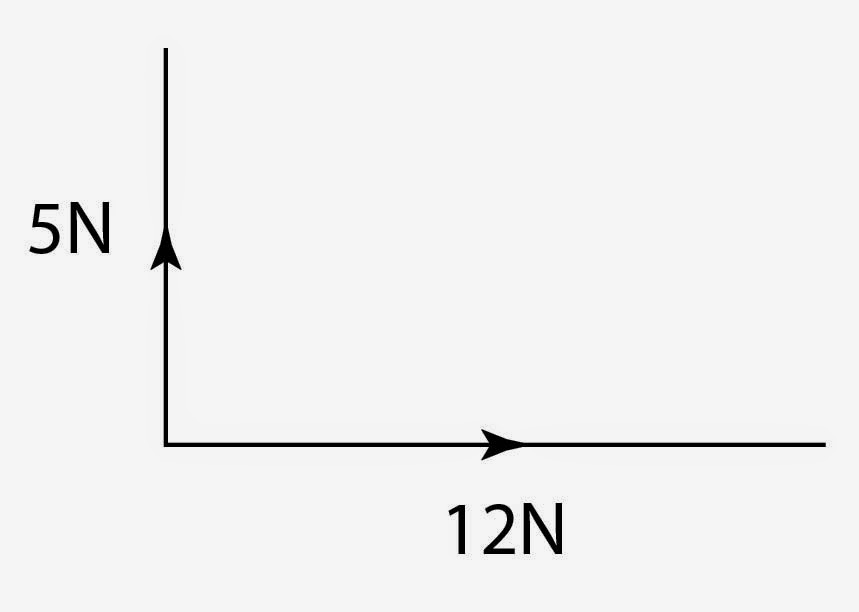# Resultant Forces

## Resultant Force

As mentioned in a previous lesson, the resultant force is the overall force acting on an object. This is where all the forces can be replaced by a single force that has the exact same effect as all the original forces acting together.

## Describing Resultant Forces

As mentioned previously we have already look at two cases and the descriptions of resultant forces. We need to discuss what happens if all of the forces are balanced, which means the resultant force is 0N. If this is the case, where there is no resultant force, we can say that the object is moving at a constant velocity. To phrase another way, the object is either moving at a constant speed or is stationary (not moving).

## Drawing Resultant Forces

Some of the more complicated resultant forces occur when the forces are acting at right angles to each other.In the example here, the resultant force is a diagonal force pointing top right..

To find the value of this force you have to draw these forces to scale!

For example, the 5N might be 5cm and the 12N is 12cm.

We then draw on of the forces, say the 12N and then we draw the 5N going upwards.

You can then measure the distance between the the start of the 12N line and the end of the 5N line to get your answer. This means that you MUST bring a ruler to the exam!!

The same can be done the other way around. If you are given a diagonal line and are asked to write down the vertical and the horizontal components of the vector then draw the respective lines and measure them. Take heed of any scale factor that is given!

Find the resultant force for an object which has a vertical component upwards of 5N and a horizontal component of 8N to the left.You will need to draw them out to scale.